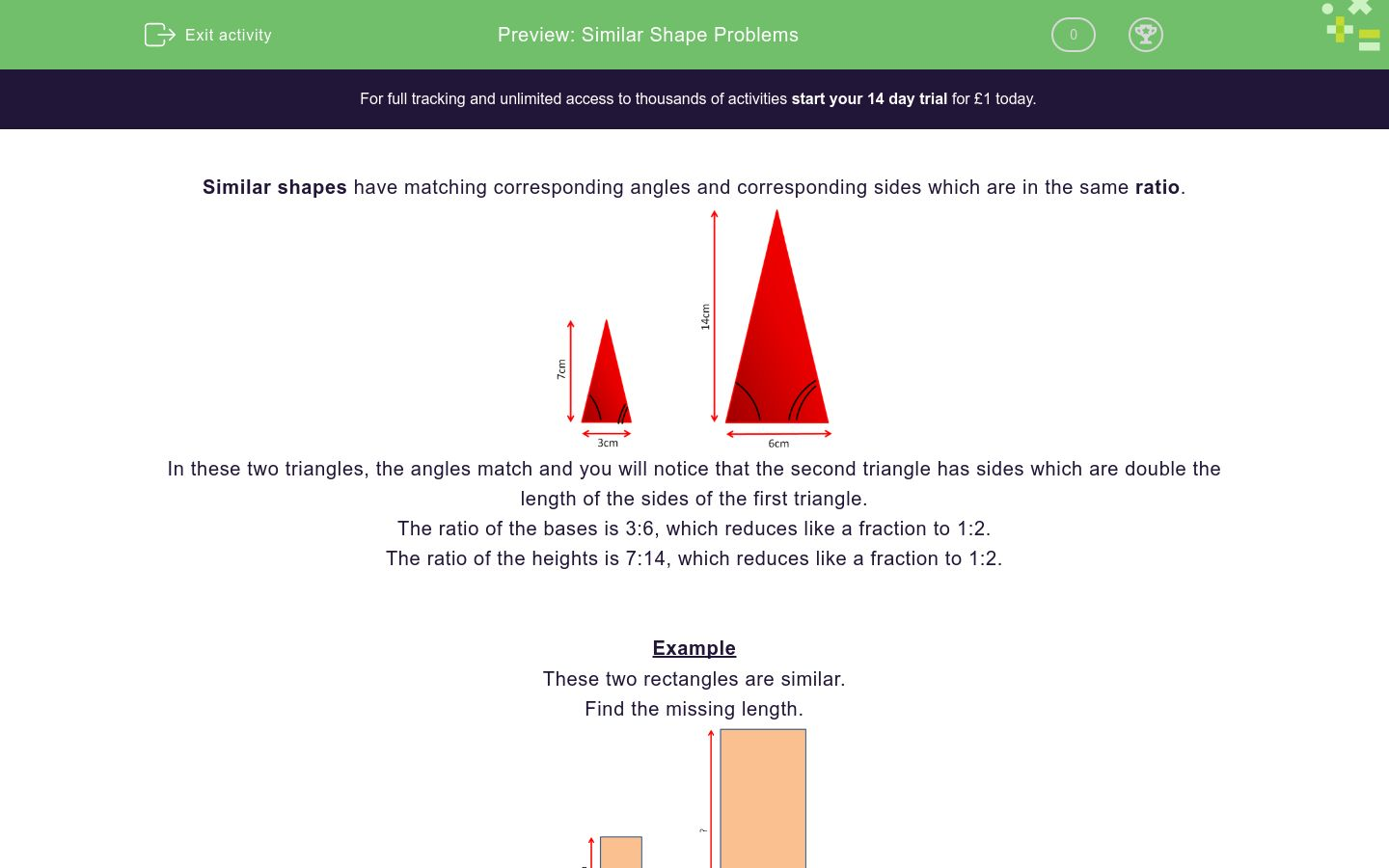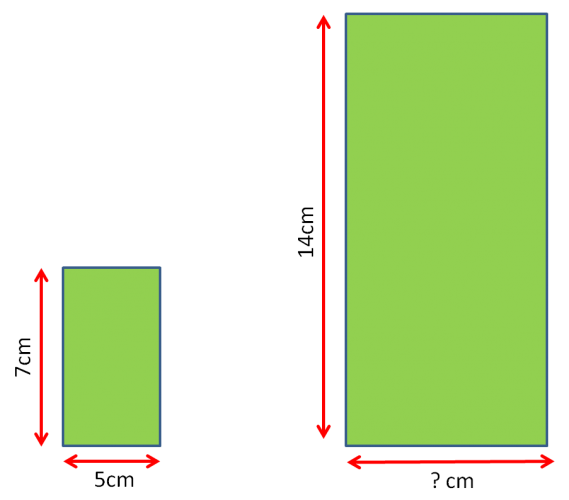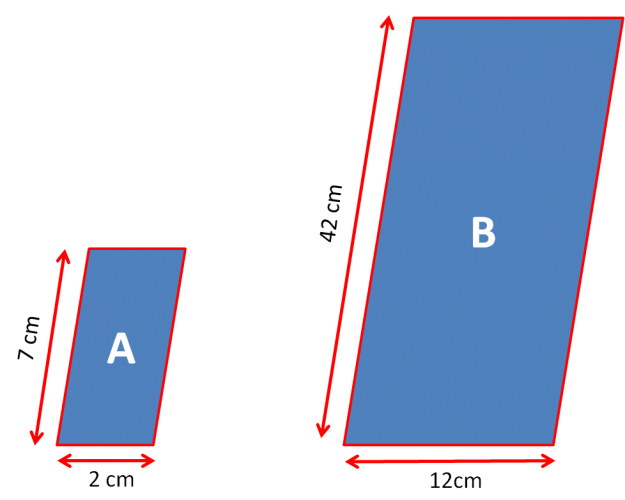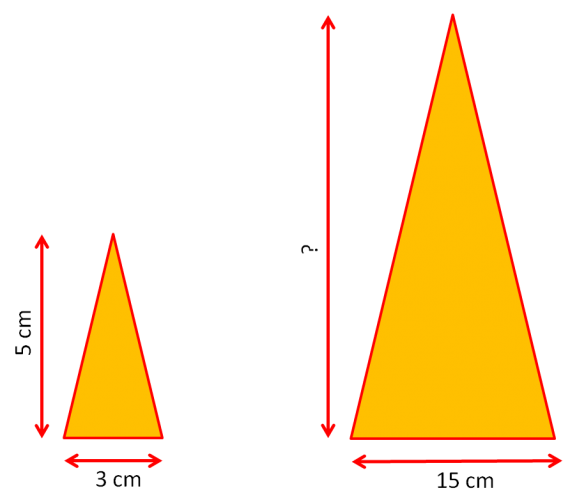# Similar Shape Problems

In this worksheet, students solve problems involving similar shapes where the scale factor is known or can be found.Key stage:  KS 2

Curriculum topic:   Ratio and Proportion

Curriculum subtopic:   Solve Shape Problems

Difficulty level:### QUESTION 1 of 10

Similar shapes have matching corresponding angles and corresponding sides which are in the same ratio.In these two triangles, the angles match and you will notice that the second triangle has sides which are double the length of the sides of the first triangle.

The ratio of the bases is 3:6, which reduces like a fraction to 1:2.

The ratio of the heights is 7:14, which reduces like a fraction to 1:2.

Example

These two rectangles are similar.

Find the missing length.The ratio of the bases is 4:8, which reduces to 1:2.

The big rectangle's sides are 2 times the matching sides of the small rectangle.

The missing side is 2 × 5 = 10 cm.

Look at these similar rectangles.

What is the ratio of their lengths, reduced to its lowest terms?5:10

1:2

3:6

10:5

Look at these similar rectangles.

What is the ratio of their lengths, reduced to its lowest terms?These two shapes are similar.

State the missing length in cm.

(Just write the number.)These two shapes are similar.

State the missing length in cm.

(Just write the number.)These two shapes are similar.

State the missing length in cm.

(Just write the number.)These two shapes are similar.

State the missing length in cm.

(Just write the number.)These two shapes are similar.

State the missing length in cm.

(Just write the number.)These two shapes are similar.

What is the ratio of their lengths, reduced to its lowest terms?These two shapes are similar.

State the missing length in cm.

(Just write the number.)These two shapes are similar.

State the missing length in cm.

(Just write the number.)• Question 1

Look at these similar rectangles.

What is the ratio of their lengths, reduced to its lowest terms?1:2
EDDIE SAYS
3:6 and 5:10 reduce like a fraction to 1:2.
• Question 2

Look at these similar rectangles.

What is the ratio of their lengths, reduced to its lowest terms?1:2
EDDIE SAYS
7:14 and 5:10 reduce like a fraction to 1:2.
• Question 3

These two shapes are similar.

State the missing length in cm.

(Just write the number.)5
EDDIE SAYS
Ratio is 1:2, so missing length is half of 10.
• Question 4

These two shapes are similar.

State the missing length in cm.

(Just write the number.)2
EDDIE SAYS
Ratio is 1:4, so missing length is a quarter of 8.
• Question 5

These two shapes are similar.

State the missing length in cm.

(Just write the number.)21
EDDIE SAYS
Ratio is 1:3, so missing length is 7 × 3 = 21.
• Question 6

These two shapes are similar.

State the missing length in cm.

(Just write the number.)3
EDDIE SAYS
Ratio is 1:5, so missing length is 15 ÷ 5 = 3.
• Question 7

These two shapes are similar.

State the missing length in cm.

(Just write the number.)10
EDDIE SAYS
Ratio is 1:2, so missing length is double 5.
• Question 8

These two shapes are similar.

What is the ratio of their lengths, reduced to its lowest terms?1:6
EDDIE SAYS
2:12 and 7:42 reduce to 1:6 like fractions.
• Question 9

These two shapes are similar.

State the missing length in cm.

(Just write the number.)25
EDDIE SAYS
Ratio is 1:5, so missing length is 5 × 5 = 25.
• Question 10

These two shapes are similar.

State the missing length in cm.

(Just write the number.)21
EDDIE SAYS
Ratio is 1:3, so missing length is 3 × 7 = 21.
---- OR ----

Sign up for a £1 trial so you can track and measure your child's progress on this activity.

### What is EdPlace?

We're your National Curriculum aligned online education content provider helping each child succeed in English, maths and science from year 1 to GCSE. With an EdPlace account you’ll be able to track and measure progress, helping each child achieve their best. We build confidence and attainment by personalising each child’s learning at a level that suits them.

Get started